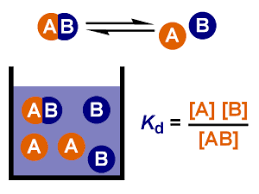# Dissociation Equation Calculator

Created by Gabriela Diaz
Last updated: Jul 01, 2022

The dissociation equation calculator will help you calculate the dissociation constant $K_d$ and the association constant $K_a$. By introducing the chemical equilibrium principle, we'll show how to obtain the dissociation equation and its reciprocal, the association.

Certain chemical reactions are reversible. Constantly converting reactants into products and vice-versa. This is the case of dissociation and association processes. Molecules of substances dissociate in aqueous solutions into atoms, ions, or radicals and then back into large molecules.

• The general chemical equilibrium constant $K$ and how to derive it; and
• The specific case of the dissociation constant $K_d$ for aqueous solutions.

## Chemical equilibrium constant K - Equilibrium constant expression

Some chemical reactions are reversible. This is, the conversion of reactants to products and products to reactants occurring simultaneously. Since the source of reactants for both reactions is constantly being renewed, the reaction never ceases. These types of systems are described as dynamic. The dynamic nature of a reversible reaction is made clear by considering the reaction rate constants for the left and right processes by the law of mass action.

Let's study the relationship between equilibrium and rates of reaction for the general equation that takes place in a single step, both to the right and to the left:

$\text{A} + \text{B} \rightleftharpoons \text{C} + \text{D}$

From here the rates of right and left reactions can be written as:

$v_{1} = k_1 \, [\, \text{A}\, ][\, \text{B}\, ] \\ v_{2} = k_2 \, [\, \text{C}\, ][\, \text{D}\, ]$

where:

• $v_1$ and $v_2$ - Chemical reaction rates for left to right and right to left reactions;
• $k_1$ and $k_2$ - Proportionality constants for both sides reactions;
• $[\ \text{A}\ ]$ and $[\ \text{B}\ ]$ - Molar concentrations of the reactants; and
• $[\ \text{C}\ ]$ and $[\ \text{D}\ ]$ - Molar concentrations of the products.

In equilibrium, reactions rates for both sides are the same:

$k_1 \, [\, \text{A}\, ][\, \text{B}\, ] = k_2 \, [\, \text{C}\, ][\, \text{D}\, ]$

By rearranging, leaving products in the numerator and reactants in the denominator, we can obtain the chemical equilibrium constant expression:

\begin{aligned} K &= \cfrac{[\ \text{products} \ ]}{[\ \text{reactants} \ ]} \\ K &= \cfrac{k_1}{k_2} = \cfrac{[\, \text{C}\, ][\, \text{D}\, ]}{[\, \text{A}\, ][\, \text{B}\, ]} \\ \end{aligned}

Where $K$ represents the equilibrium constant for a simple reversible process, relating the rate of chemical reaction constants on the right and on the left. This is also known as the law of mass action.

The above corresponds to the case where stoichiometric coefficients are equal to the unit, but let's see which would be the expression for a generic chemical reaction:

$a\text{A} + b\text{B} + \cdots \ \rightleftharpoons \ c\text{C} + d\text{D} + \cdots$

Where $a$, $b$, $c$, and $d$ are the stoichiometric coefficients that balance the reaction. Then, the equilibrium constant $K$ is then represented as:

$K = \cfrac{[\, \text{C}\, ]^c[\, \text{D}\, ]^d\cdots}{[\, \text{A}\, ]^a[\, \text{B}\, ]^b\cdots}$

This constant should always be a positive value. Different values indicate the tendency of the direction of the reaction. When:

• $K$ > 1 - The reaction moves to the right, and there are more products than reactants.
• $K$ = 1 - The molarities of the reactants and products are about the same; and
• $K$ < 1 - The reaction moves to the left, and there are more reactants than products.

💡 The equilibrium constant $K$ is also affected by temperature and pressure.

## Dissociation constant Kd

Dissociation is the process by which a substance breaks down into smaller parts, as is the case for complexes into molecules or a molecule of salt into ions when dissolved in water in a reversible way.

The dissociation constant $K_d$ is a specific type of equilibrium constant (law of mass action), that quantifies the tendency of a substance to reversibly dissociate. The higher the value of $K_d$, the greater the tendency to dissociate. In the specific case of salts, the dissociation constant is also known as the ionization constant.

The general equation for the dissolution of a substance in water is:

$\text{AB}_{(aq)} + \text{H}_2\text{O}_{(l)} \rightleftharpoons \text{A}_{(aq)} + \text{B}_{(aq)}$

Similarly to the general equilibrium constant deduction, the dissociation constant $K_d$ formula can be expressed as:

\begin{aligned} K_d &= \cfrac{[\, \text{A}\, ][\, \text{B}\, ]}{[\, \text{AB}\, ][\, \text{H}_2\text{O}\, ]} \\ K_d &= \cfrac{[\, \text{A}\, ][\, \text{B}\, ]}{[\, \text{AB}\, ][\, 1\, ]} \\ \end{aligned}

Because the water is assumed to be pure, its concentration is represented as constant with a value of 1. As a result, the dissociation constant is as follows:

$K_d = \cfrac{[\, \text{A}\, ][\, \text{B}\, ]}{[\, \text{AB}\, ]}$

This is the equation that the dissociation equation calculator uses.

The units of the dissociation constant are in the molarity units or molar concentration (1 M = 1 mol/liter).

The dissociation constant is the inverse of the association constant $K_a$:

$K_a = \cfrac{1}{K_d}$

For weak acids and bases, the dissociation constant is expressed as $K_a$ and $K_b$ respectively. With the acid dissociation constant you can calculate the pH of a buffer solution.

Why not take a look at our solution dilution calculator? This tool will help you determine the final concentration of a solution that you're diluting

Gabriela DiazConcentration of A [A]
M
Concentration of B [B]
M
Concentration of AB [AB]
M
Dissociation constant (Kd)
M
Association constant (Ka)
1/
M
People also viewed…

### FRET efficiency

With our FRET efficiency calculator you learn how to calculate both the critical distance and the efficiency of this useful non-radiative process.

### Mole

Our mole calculator is an invaluable tool for your chemistry homework!